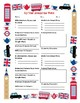# Real-world Math with Google ToursSubject
Resource Type
File Type

PDF

(1 MB|4 pages)
Standards
• Product Description
• Standards
Get your students going on a Google Tour to develop fluency with adding, subtracting, multiplying and dividing multi-digit decimals using the standard algorithm for each operation (6.NS.B.3).

This template will allow your students to organize and create a Google Tour and work with multi-digit numbers and decimals. Students can apply algorithms to multi-digit numbers and hit the math practice standards
*Reason abstractly and quantitatively and Look for and express regularity in repeated reason. (SMP 2 & 8)

Best of all this is an interactive math lesson that allows for differentiation without recreating the lesson. Your students will have fun exploring the world and you can easily tie in other math standards that focus on percent, geometry, measurement, and ratios.

You decide what your students are ready for! Check out my blog for additional ideas on this lesson and videos to support you in Google Tours: https://makingmathconnection.blogspot.com/
Understand that positive and negative numbers are used together to describe quantities having opposite directions or values (e.g., temperature above/below zero, elevation above/below sea level, credits/debits, positive/negative electric charge); use positive and negative numbers to represent quantities in real-world contexts, explaining the meaning of 0 in each situation.
Fluently add, subtract, multiply, and divide multi-digit decimals using the standard algorithm for each operation.
Fluently divide multi-digit numbers using the standard algorithm.
Total Pages
4 pages
N/A
Teaching Duration
50 minutes
Report this Resource to TpT
Reported resources will be reviewed by our team. Report this resource to let us know if this resource violates TpT’s content guidelines.